Homework Help Question & Answers

# The utility department of Brentwood City needs you to write a C++ program that will print...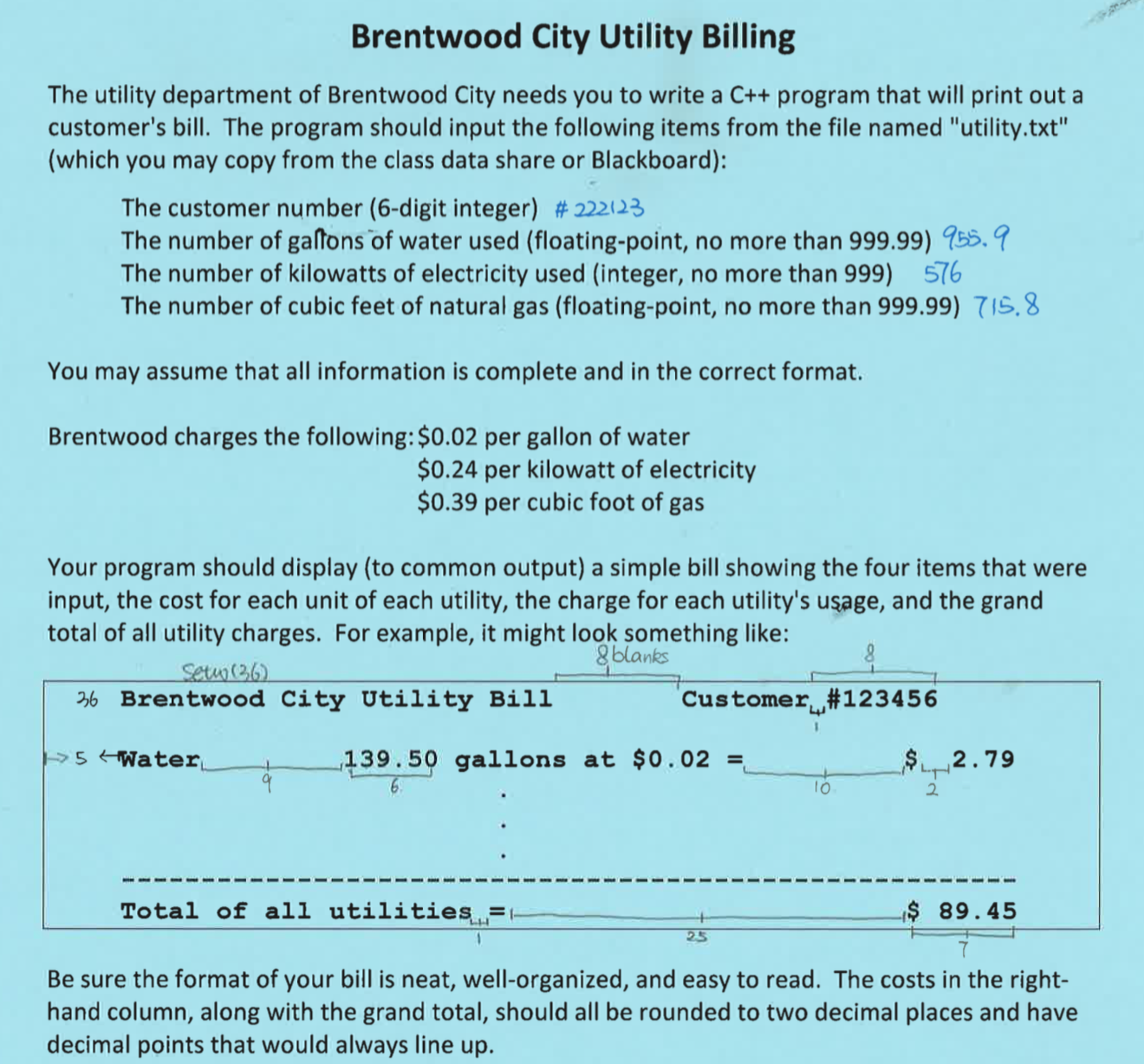The utility department of Brentwood City needs you to write a C++ program that will print out a customer's bill. The program should input the following items from the file named "utility.txt" (which you may copy from the class data share or Blackboard): The customer number (6-digit integer) The number of gallons of water used (floating-point, no more than 999.99) The number of kilowatts of electricity used (integer, no more than 999) The number of cubic feet of natural gas (floating-point, no more than 999.99) You may assume that all information is complete and in the correct format. Brentwood charges the following: \$0.02 per gallon of water \$0.24 per kilowatt of electricity \$0.39 per cubic foot of gas Your program should display (to common output) a simple bill showing the four items that were input, the cost for each unit of each utility, the charge for each utility's usage, and the grand total of all utility charges. For example, it might look something like: Be sure the format of your bill is neat, well-organized, and easy to read. The costs in the right-hand column, along with the grand total, should all be rounded to two decimal places and have decimal points that would always line up.

#### Homework Answers

Answer #1

utility.txt(Save the text file in D:Drive .Then the path of the file is D:\\utility.txt )

222123
955.9
576
715.8

____________________________________________

Code in c++

#include <fstream>
#include <iostream>
#include <iomanip>
using namespace std;

int main() {
//Declaring constant
const char* filename = "D:\\utility.txt";

//Declaring variables
long cust_number,cnum;
float gallons_water,gwater;
int electricity_kw,electricity;
float natural_gas_cf,gas;
float const WATER=0.02,ELEC=0.24,GAS=0.39;

//Input stream
std::ifstream inFile(filename);

//Check whether the file is available or not.
if(!inFile) {
cout << endl << "Failed to open file " << filename;
return 1;
}

//If the file is available,read the values from it.
while(!inFile.eof()) {
inFile >>cnum>>gwater>>electricity>>gas;
cust_number=cnum;
gallons_water=gwater;
electricity_kw=electricity;
natural_gas_cf=gas;

//calculating the cost
float cost_of_water=gallons_water*WATER;
float cost_of_electricity=electricity_kw*ELEC;
float cost_of_gas=natural_gas_cf*GAS;

//calculating the total Cost.
float total_charges=cost_of_water+cost_of_electricity+cost_of_gas;

//Displaying the Bill.
cout << std::setw(36) << "Brentwood City Utility Bill"<<setw(16)<<"Customer #"<<cust_number<<endl<<"\n";
cout<<std::setw(14)<<"Water"<<setw(17)<<gallons_water<<" gallons at \$"<<WATER<<"="<<setw(14)<<"\$"<<setw(2)<<cost_of_water<<endl;
cout<<std::setw(20)<<"Electricity"<<setw(9)<<electricity_kw<<" Kilowatts at \$"<<ELEC<<"="<<setw(14)<<"\$"<<setw(2)<<cost_of_electricity<<endl;
cout<<std::setw(20)<<"Natural Gas"<<setw(11)<<natural_gas_cf<<" cubic foot at \$"<<GAS<<"="<<setw(11)<<"\$"<<std::fixed<< std::setprecision(2)<<setw(2)<<cost_of_gas<<endl<<"\n";
cout<<std::setw(69)<<"------------------------------------------------------------"<<endl;
cout<<std::setw(33)<<"Total of all utilities ="<<setw(30)<<"\$ "<<total_charges<<endl;
}
cout << endl;
return 0;
}

________________________________________________

Output: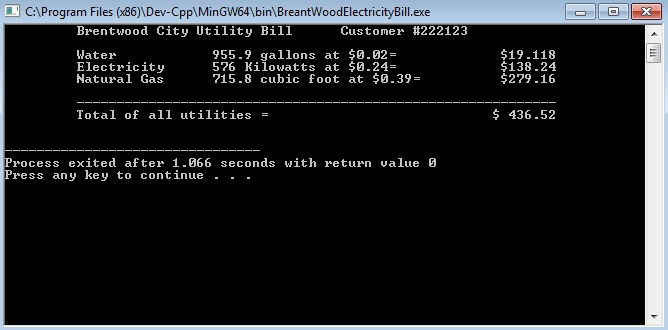Know the answer?
Your Answer:

#### Post as a guest

Your Name:

What's your source?

#### Earn Coin

Coins can be redeemed for fabulous gifts.

Not the answer you're looking for? Ask your own homework help question. Our experts will answer your question WITHIN MINUTES for Free.
Similar Homework Help Questions
• ### INSTRUCTION AND PROBLEMS Write a Python program for each of the problems in this lab. Please...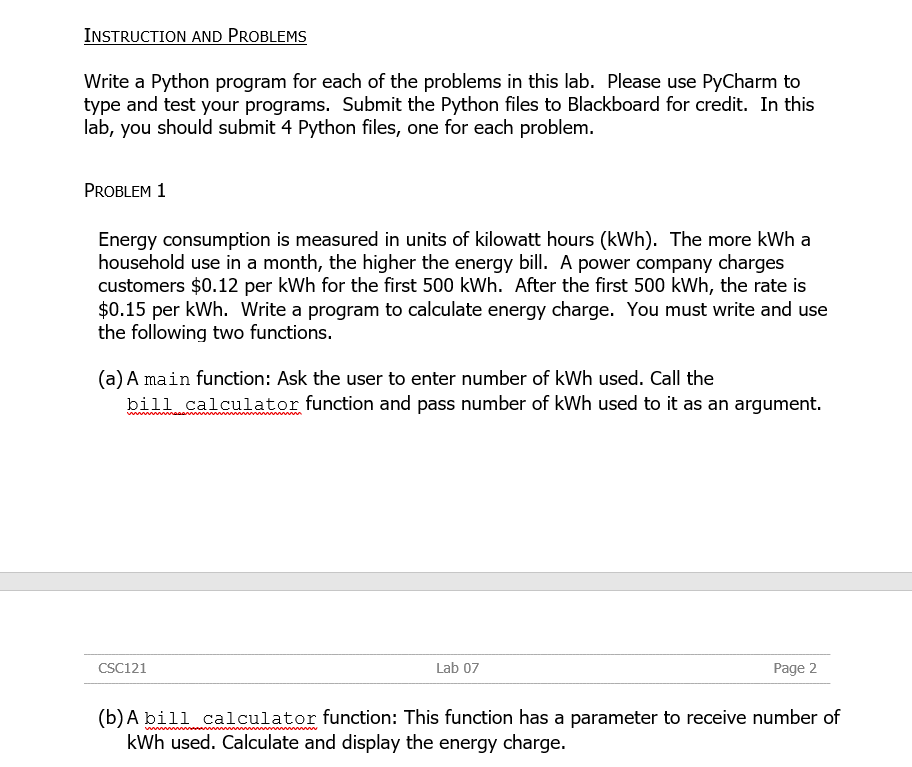INSTRUCTION AND PROBLEMS Write a Python program for each of the problems in this lab. Please use PyCharm to type and test your programs. Submit the Python files to Blackboard for credit. In this lab, you should submit 4 Python files, one for each problem PROBLEM I Energy consumption is measured in units of kilowatt hours (kWh). The more kWh a household use in a month, the higher the energy bll. A power company charges customers \$0.12 per kWh for...

• ### WRITE A PROGRAM IN C THAT DOES THE FOLLOWING: The numbers are real numbers, read in...

WRITE A PROGRAM IN C THAT DOES THE FOLLOWING: The numbers are real numbers, read in as double precision floating-point numbers, one number perline, possibly with some white space before or after. Hint: use scanf. There are guaranteed to be no more than one million (that’s 10^6) numbers in the input, and no fewer than two legitimate numbers. Any number that is equal to zero should be ignored (discarded, not stored in the array or counted in any way).

• ### gas meter problem in C++

This is a “gas meter” problem. The gas meters in the problem show integer values only in the range of 0 to 9999 cubic meters. When the meter reaches 9999, it then“starts over” with the value 0000. This is a common consideration that has to be handled anytime we measure things, since all measurement devices place a limit onthe largest number the device can record.Problem statementSuppose charges by a gas company are based on the user’s consumption, according to the...

• ### //I NEED THE PROGRAM IN C LANGUAGE!// QUESTION: I need you to write a program which...

//I NEED THE PROGRAM IN C LANGUAGE!// QUESTION: I need you to write a program which manipulates text from an input file using the string library. Your program will accept command line arguments for the input and output file names as well as a list of blacklisted words. There are two major features in this programming: 1. Given an input file with text and a list of words, find and replace every use of these blacklisted words with the string...

• ### THE PROBLEM: The nice folks from the Lion Lending Company have hired you to write some...

THE PROBLEM: The nice folks from the Lion Lending Company have hired you to write some software that will process their daily loan information. The data will come from a text file that has the following format: The first line will contain an integer: number of loan applications There will be two rows for each of the customers First line will be the customer's (1) first name and (2) last name, separated by a space (assume there is no spaces...

• ### Write a program **(IN C)** that displays all the phone numbers in a file that match the area code...

Write a program **(IN C)** that displays all the phone numbers in a file that match the area code that the user is searching for. The program prompts the user to enter the phone number and the name of a file. The program writes the matching phone numbers to the output file. For example, Enter the file name: phone_numbers.txt Enter the area code: 813 Output: encoded words are written to file: 813_phone_numbers.txt The program reads the content of the file...

• ### Write a C++ program that creates the following eight two dimensional random number vector: (a) the...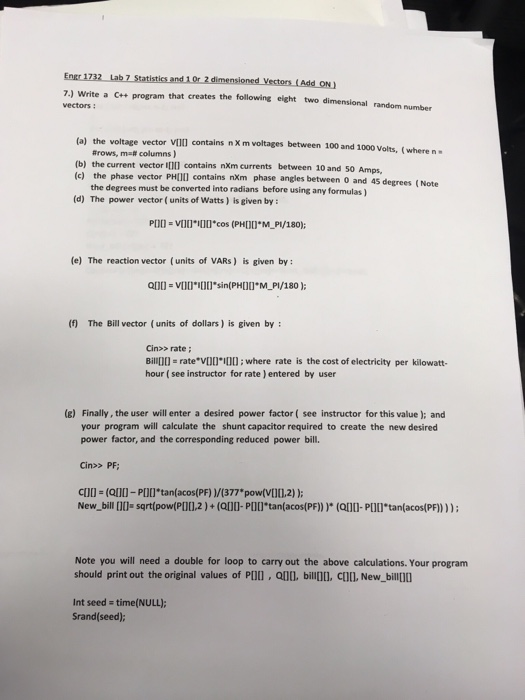Write a C++ program that creates the following eight two dimensional random number vector: (a) the voltage vector V[][] contains n X m voltages between 100 and 1000 Volts, (where n.= #rows, m=# columns) (b) the current vector I[][] contains nXm currents between 10 and 50 Amps, (c) the phase vector PH[][] contains nXm phase angles between 0 and 45 degrees (d) The power vector (units of Watts) is given by: P[][] = V[][]*I[]*cos(PH[][]*M_P1/180); (e) The reaction vector (units of...

• ### In this project you will write a C++ program that simulates the purchase of a single...

In this project you will write a C++ program that simulates the purchase of a single item in an online store. What to do Write a C++ program that: 1. Prints out a welcome message. 2. Prompts the user for the following information: (a) Their first name (example: Roger) (b) Their last name (example: Waters) (c) The name of the product (example: Brick) (d) The unit price of the product (example: 1.99) (e) The quantity to buy (example: 200) (f)...

• ### In Java. Write a GUI contact list application. The program should allow you to input names...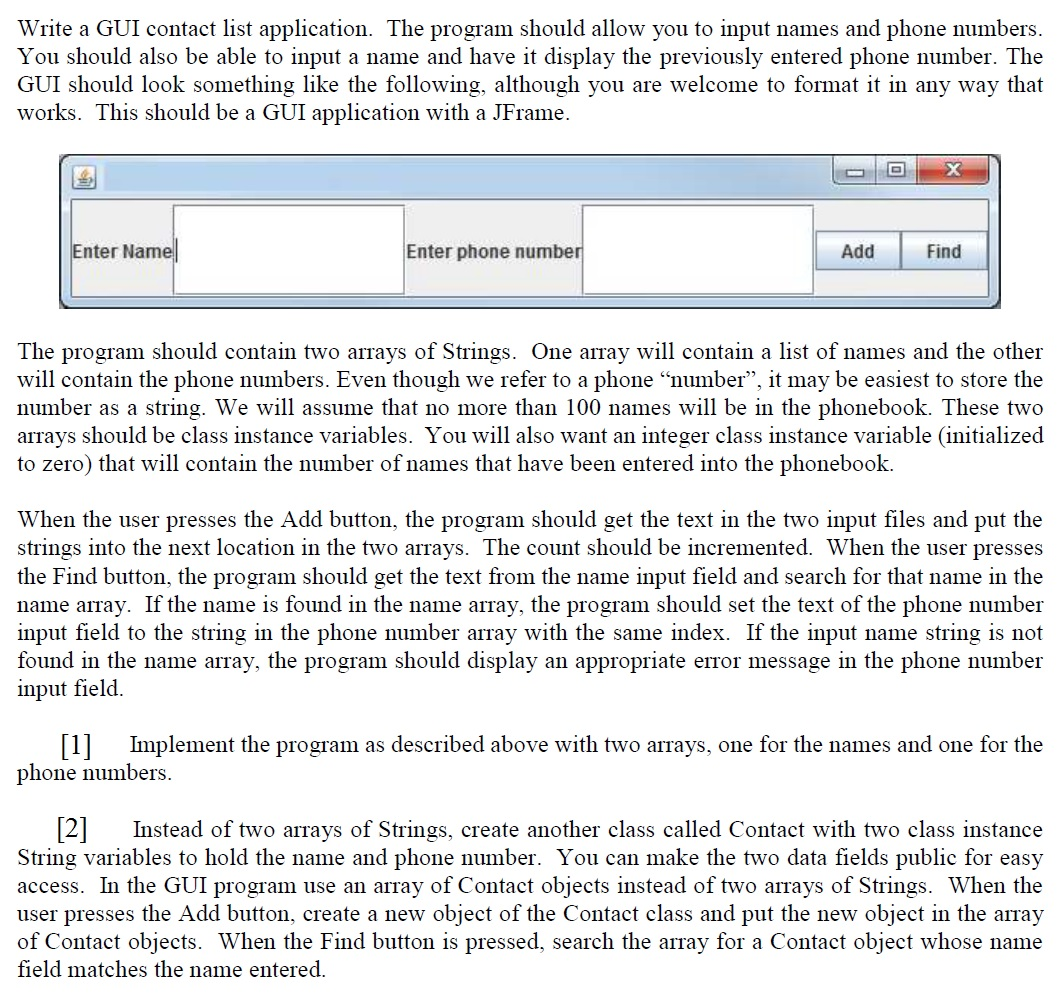In Java. Write a GUI contact list application. The program should allow you to input names and phone numbers. You should also be able to input a name and have it display the previously entered phone number. The GUI should look something like the following, although you are welcome to format it in any way that works. This should be a GUI application with a JFrame. The program should contain two arrays of Strings. One array will contain a list...

• ### c++ Write a C++ program using the struct keyword to help a local restaurant automate its...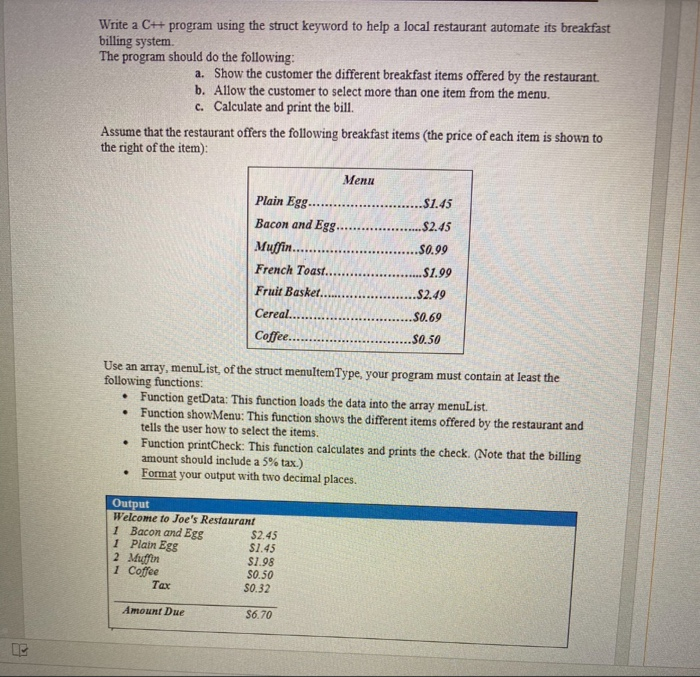c++ Write a C++ program using the struct keyword to help a local restaurant automate its breakfast billing system The program should do the following: a. Show the customer the different breakfast items offered by the restaurant. b. Allow the customer to select more than one item from the menu. c. Calculate and print the bill. Assume that the restaurant offers the following breakfast items (the price of each item is shown to the right of the item) Menu Plain...